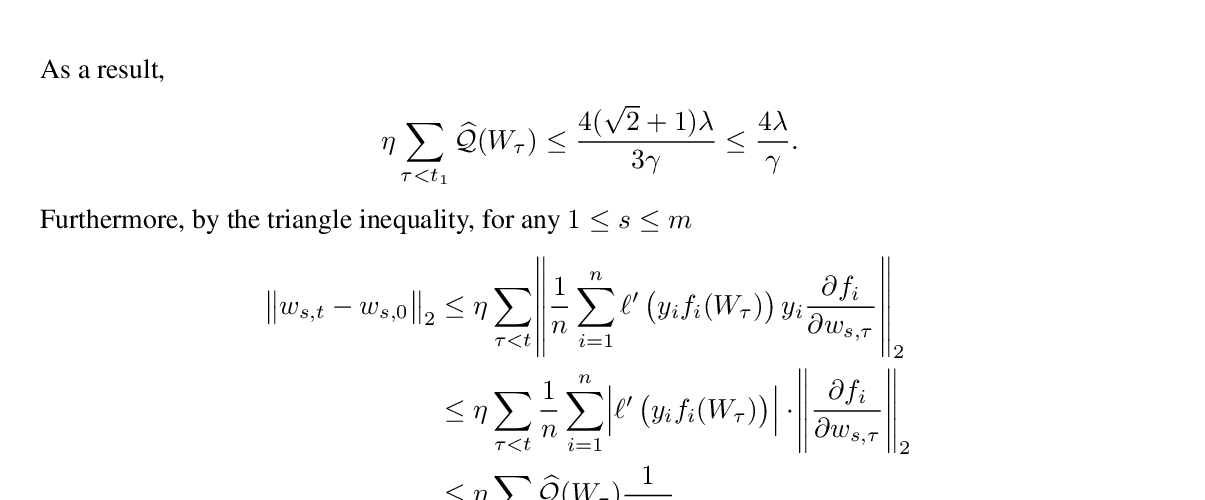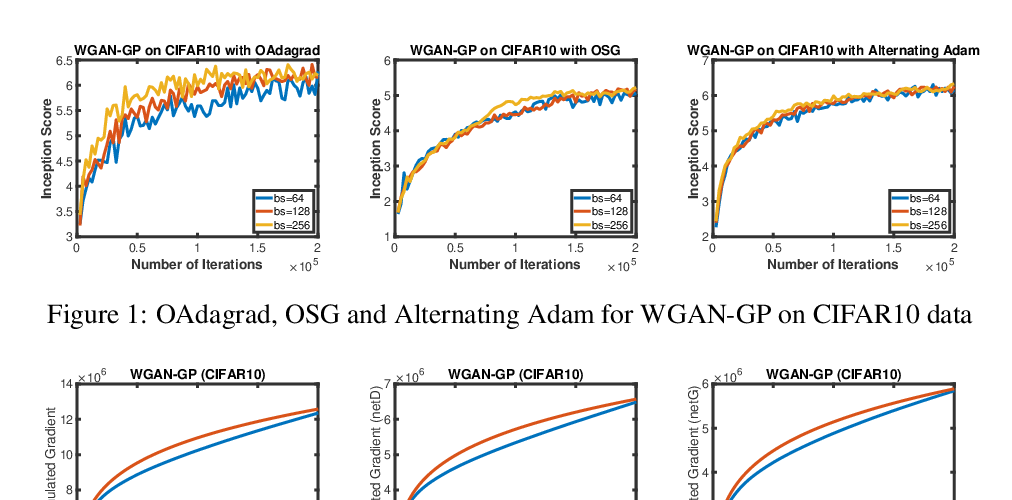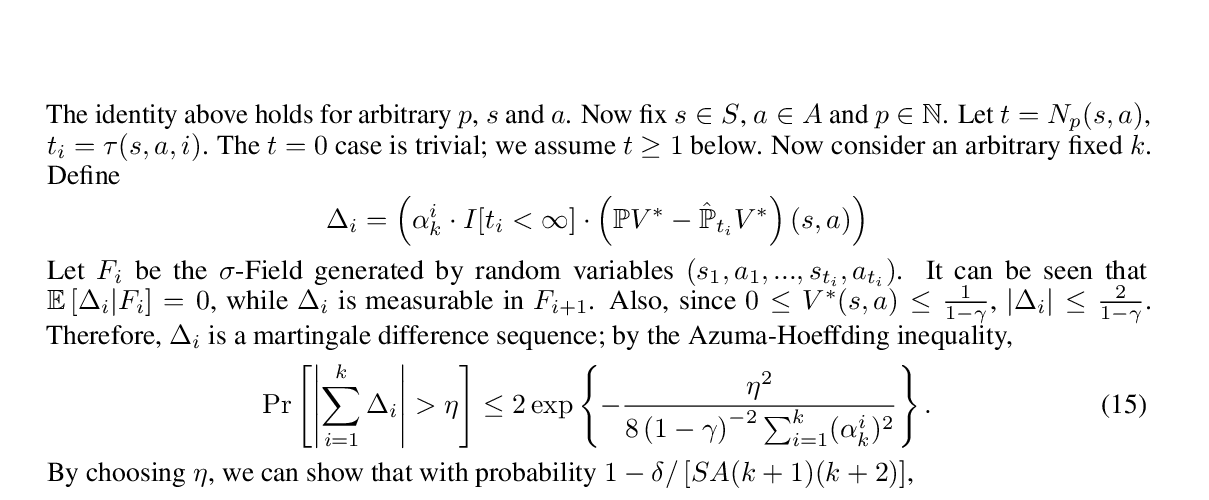## Sample Efficient Policy Gradient Methods with Recursive Variance Reduction

### Pan Xu, Felicia Gao, Quanquan Gu

Abstract: Improving the sample efficiency in reinforcement learning has been a long-standing research problem. In this work, we aim to reduce the sample complexity of existing policy gradient methods. We propose a novel policy gradient algorithm called SRVR-PG, which only requires $O(1/\epsilon^{3/2})$\footnote{$O(\cdot)$ notation hides constant factors.} episodes to find an $\epsilon$-approximate stationary point of the nonconcave performance function $J(\boldsymbol{\theta})$ (i.e., $\boldsymbol{\theta}$ such that $\|\nabla J(\boldsymbol{\theta})\|_2^2\leq\epsilon$). This sample complexity improves the existing result $O(1/\epsilon^{5/3})$ for stochastic variance reduced policy gradient algorithms by a factor of $O(1/\epsilon^{1/6})$. In addition, we also propose a variant of SRVR-PG with parameter exploration, which explores the initial policy parameter from a prior probability distribution. We conduct numerical experiments on classic control problems in reinforcement learning to validate the performance of our proposed algorithms.

## Similar Papers

##### Polylogarithmic width suffices for gradient descent to achieve arbitrarily small test error with shallow ReLU networks
###### Ziwei Ji, Matus Telgarsky,##### Towards Better Understanding of Adaptive Gradient Algorithms in Generative Adversarial Nets
###### Mingrui Liu, Youssef Mroueh, Jerret Ross, Wei Zhang, Xiaodong Cui, Payel Das, Tianbao Yang,##### Q-learning with UCB Exploration is Sample Efficient for Infinite-Horizon MDP
###### Yuanhao Wang, Kefan Dong, Xiaoyu Chen, Liwei Wang,